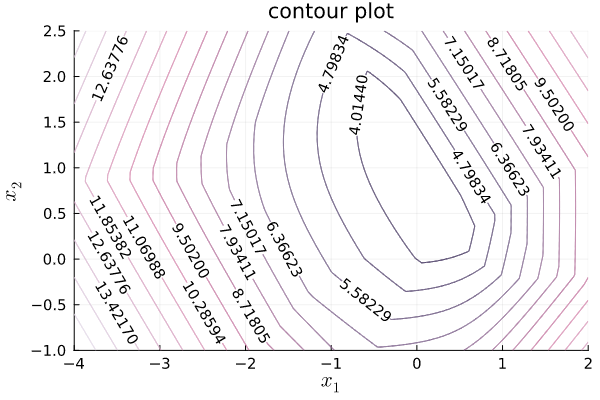# Plots.contour rounding the contour labels

I am using `Plots.jl` to generate a contour plot. It works great but the contour labels have 5 digits after the decimal point:How can I update my code, which is shared next, so that the contour labels are rounded to decimals? I looked into this for a while, and there is no easy way so far. I would prefer an option that does not use any other visualisation package than `Plots.jl`. Thank you.

``````x_ran = range(-4, 2.0, length=600)
y_ran = range(-1, 2.5, length=600)
z = @. eval_f(x_ran', y_ran)
Plots.contour(x_ran, y_ran, z, levels=15,
color=:acton, xaxis = (L"x_1",font(10)),
yaxis = (L"x_2", font(10)), clabels= true,
title = "contour plot",
cbar=false, lw=1,
xguidefontsize=14, yguidefontsize=14)
``````

For your example, try passing to `contour()` the keyword argument:
`levels=4:13`

1 Like

Thanks! Good hack!

In Makie you can pass a labelformatter:

``````using CairoMakie, Printf
using Makie.LaTeXStrings
eval_f(x, y) = cos(x) * sin(y)
x_ran = range(-4, 2.0, length=600)
y_ran = range(-1, 2.5, length=600)
z = @. eval_f(x_ran', y_ran)

contour(x_ran, y_ran, z, levels=15,
labels=true, labelformatter=(x) -> @sprintf("%.1f", x),
colormap=:acton, axis=(xlabel=L"x_1", ylabel=L"x_2", title="contour plot"),
)
``````

7 Likes